Mobile QR CodeBootstrap, CMOS, fully differential, mirrored-cascode, TIA

## I. INTRODUCTION

Recently, wireless sensor networks (WSNs) have been attracting great attention because of many potential applications: healthcare, manufacturing, environmental monitoring, and agriculture (1-3). In WSNs, a large number of wireless sensor terminals are installed to gather various kinds of sensing data: temperature, pressure, acceleration, and so on. Increasing the number of the sensor terminals working for WSN applications, however, causes increasing the total power consumption. Therefore, reducing power consumption of each RF component consisting of the terminals is necessary.

A low-noise amplifier (LNA) is the first active block of the RF front-end in a wireless receiver (4,5). In WSN applications, low power consumption, wide bandwidth, and small chip area are required. In general, LNAs using on-chip inductors obtain gain and high-frequency operation because of the resonance characteristics. On the other hand, the frequency bandwidth is much narrower than other types of amplifiers. Also, it is not suited for miniaturization because the inductors occupy huge area on a CMOS chip. Inductorless amplifiers achieve wide bandwidth while occupying small area, although their gain is not so high. For these reasons, inductorless amplifiers are preferable from the viewpoint of saving chip area.

Various topology of inductorless LNAs has been proposed and developed (6-15). For examples, a resistive shunt-feedback type (7), an inverter-based type (8), common-gate (CG) types using the transconductance enhancement technique (9,14) were reported. As a candidate of LNAs for WSNs, an active-shunt-feedback type LNA was reported (11). In this LNA, both low power consumption (0.4 mW) and the occupation of small chip area (0.0052 mm$^{2}$) were achieved by utilizing current-reuse and active-shunt-feedback techniques in a 0.13-μm CMOS chip. The obtainable bandwidth, however, was up to 2.2 GHz, so the conventional active-shunt-feedback type LNA was not available for 2.4-GHz-band applications: Wi-Fi, Bluetooth, and ZigBee. One of the possible solutions is use of a shorter-channel CMOS process (15). Although the intrinsic gain becomes lower as channel length is decreased, use of the shorter-channel CMOS leads to reduction of the occupied chip area as well as higher frequency operation.

Fig. 2. (a) Basic structure of fabricated LNA (feedback transistor and coupling capacitor are removed from original LNA), (b) and (c) are schematic diagrams of NMOS and PMOS amplifiers corresponding to upper and lower part of (a), respectively.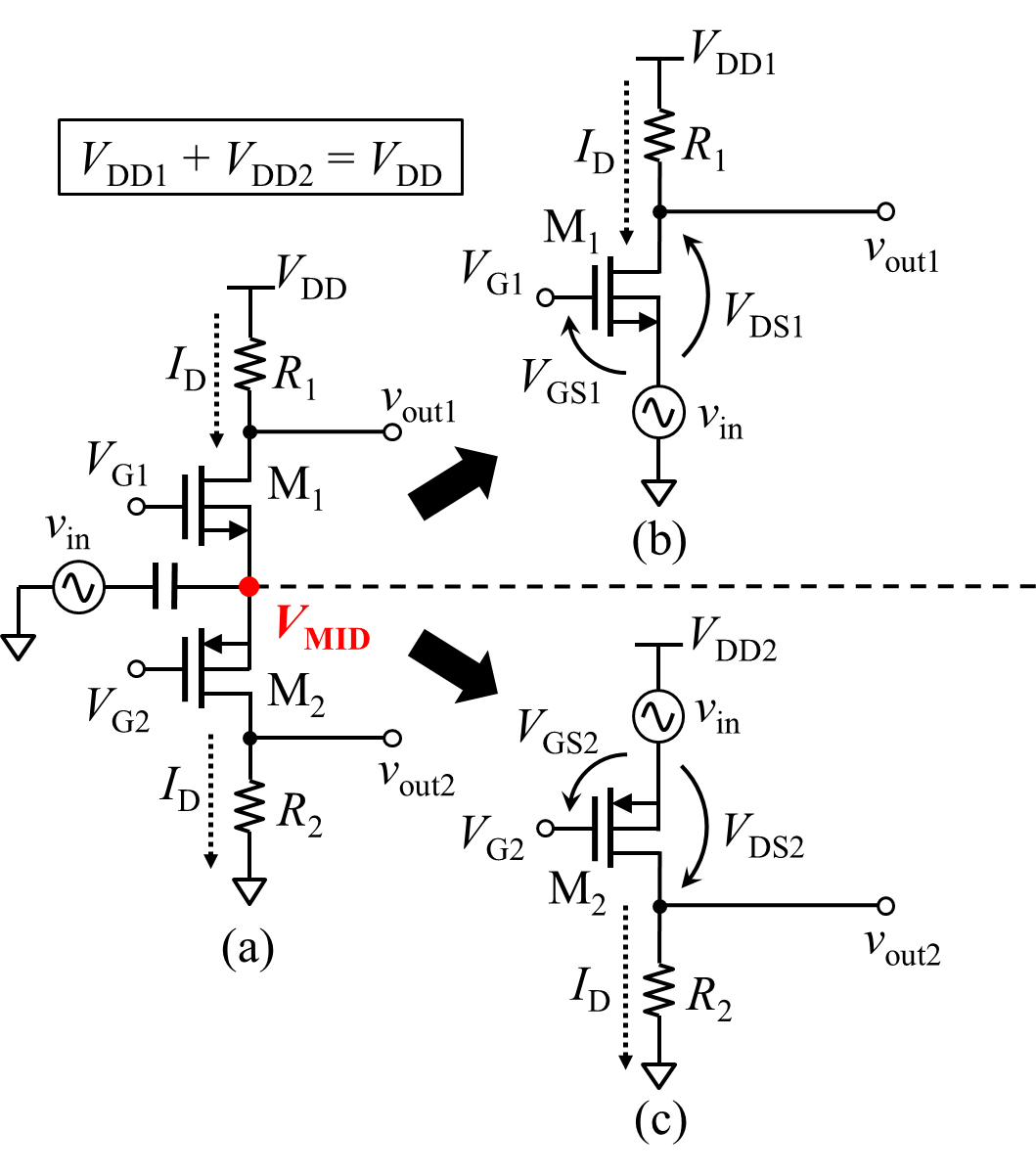In this paper, we demonstrate an active- shunt-feedback type LNA using a 65-nm CMOS process. We propose a design method focusing on the intermediate-node voltage in the LNA, and we clarify the influence of the intermediate voltage upon the gain and noise characteristics. The coupling capacitance for effective noise-cancelling is examined by circuit simulations. On the basis of our design method, the active-shunt-feedback type LNA is fabricated in a 65-nm CMOS chip, and its characteristics are evaluated. It is confirmed that our LNA has better figure-of-merit in comparison with the conventional types for WSNs.

This paper is organized as follows. In section II, the circuit configuration and operating principle are presented, and we point out how important the intermediate-node voltage is for designing the focused LNA. Section III shows analysis and simulation results based on the proposed method. Section IV and V show the measurement results of the fabricated LNA and conclusions.

## II. CIRCUIT DESCRIPTION

A schematic diagram of the fabricated LNA is shown in Fig. 1. This LNA is composed of two CG amplifiers using NMOS (M$_{1}$) and PMOS (M$_{2}$), a common-source (CS) NMOS (M$_{3}$) for active-shunt-feedback, and a capacitor ($\textit{C}$$_{\mathrm{C}}) between nodes 1 and 2 for noise-cancelling. The feedback transistor works to decrease the input impedance and enlarge the bandwidth. Also, a back-gate voltage for the feedback transistor, \textit{V}$$_{\mathrm{BG}}$, enables to change the amount of feedback current and adjust the input impedance to source impedance, $\textit{R}$$_{\mathrm{S}} (50~\Omega in most cases). The noise voltage at node 3 derived from the noise current of M_{1} and M_{2} is amplified by the other MOSFET. Since the phase of the noise voltage at node 1 is opposite to that at node 2, the coupling capacitor combines the noise voltages of nodes 1 and 2 and can be partially cancelled. ### 2. Importance of Intermediate-node Voltage Fig. 2(a) shows a basic structure of the fabricated LNA, where a feedback transistor and a coupling capacitor are removed from the original LNA shown in Fig. 1. As shown in Fig. 2(a), the focused LNA can be considered as the structure stacked with NMOS and PMOS amplifiers vertically. Hence, we can design an NMOS amplifier and a PMOS amplifier separately as shown in Fig. 2(b) and (c), where \textit{v}$$_{\mathrm{in}}$ is a small-signal voltage source; $\textit{V}$$_{\mathrm{GS}}$$_{i}$, $\textit{V}$$_{\mathrm{DS}}$$_{i}$, and $\textit{V}$$_{\mathrm{DD}}$$_{i}$ are gate-source, drain-source, and supply voltages, respectively; 1 and 2 stand for NMOS (M$_{1}$) and PMOS (M$_{2}$), respectively; $\textit{V}$$_{\mathrm{DD}}$$_{i}$ satisfies $\textit{V}$$_{\mathrm{DD1}} + \textit{V}$$_{\mathrm{DD2}}$ = $\textit{V}$$_{\mathrm{DD}}. As shown in Fig. 2(a), the drain current drawn by the NMOS and PMOS amplifiers is the almost same. Therefore, the drain current of the amplifiers, \textit{I}$$_{\mathrm{D}}$, in Fig. 2(b) and (c) should be same so that the operating condition would not be drastically changed after stacking the amplifiers.

##### (1b)
$\textit{V}$$_{\mathrm{MID}} = \textit{V}$$_{\mathrm{DD2}}$.

##### (3)
$Z_{\mathrm{in}}\cong \frac{1}{g_{\mathrm{m}1}+g_{\mathrm{m}2}}$.

To satisfy the input matching condition, $\textit{Z}$$_{\mathrm{in}} = \textit{R}$$_{\mathrm{S}}$ = 50~$\Omega$, the total transconductance, $\textit{g}$$_{\mathrm{m1}} + \textit{g}$$_{\mathrm{m2}}$, should have ~20 mS. Therefore, the input impedance is close to the matching condition when each MOSFET has ~10 mS. Note that the input matching condition does not need to be satisfied strictly in this step since a feedback transistor will be added to this structure later.

To evaluate the dependence of the intermediate-node voltage upon the characteristics of the amplifiers, we considered three cases: (i) $\textit{V}$$_{\mathrm{MID}} > \textit{V}$$_{\mathrm{DD}}$/2, (ii) $\textit{V}$$_{\mathrm{MID}} = \textit{V}$$_{\mathrm{DD}}$/2, and (iii) $\textit{V}$$_{\mathrm{MID}} < \textit{V}$$_{\mathrm{DD}}$/2. The gate-source voltage, $\textit{V}$$_{\mathrm{GS}}$$_{i}$, was set for $\textit{g}$$_{\mathrm{m}}$$_{i}$ = 10 mS, and the load resistance for each amplifier was determined as

##### (4)
$\textit{R}$$_{i} = (\textit{V}$$_{\mathrm{DD}}$$_{i} {-} \textit{V}$$_{\mathrm{DS}}$$_{i}) / \textit{I}$$_{\mathrm{D}}$.

The characteristics of the amplifiers were examined by using circuit simulators (HSPICE and Spectre) with the parameters of the TSMC 65-nm CMOS process. The supply voltage was set to $\textit{V}$$_{\mathrm{DD}} = 1.2 V; \textit{V}$$_{\mathrm{DD1}}$ and $\textit{V}$$_{\mathrm{DD2}} were set to 0.5 V and 0.7 V for the case (i), 0.6 V and 0.6~V for the case (ii), and 0.7 V and 0.5 V for the case (iii), respectively. Figs. 3-5 show the dependences of the approximate voltage gain calculated from \textit{g}$$_{\mathrm{m}}$$_{i}$$\textit{R}$$_{i} upon drain current for each case, where the filled and open symbols signify the results of NMOS and PMOS amplifiers, respectively. The data of MOSFETs operating in a saturation region are shown in Figs. 3-5. Reducing the drain current corresponds to increasing the gate width since \textit{g}$$_{\mathrm{m}}$$_{i} is fixed at 10 mS. In the case (i) shown in Fig. 3, the gains of NMOS and PMOS amplifiers are the highest at |\textit{V}$$_{\mathrm{DS1}}$| = 0.20 V and |$\textit{V}$$_{\mathrm{DS2}}| = 0.25 V, respectively. The gain of PMOS is higher than that of NMOS by ~1 dB for every \textit{I}$$_{\mathrm{D}}$. In the case (ii) shown in Fig. 4, the highest gains are obtained by setting |$\textit{V}$$_{\mathrm{DS}}$$_{i}$| = 0.20 V. The gain of NMOS amplifier is higher than that of PMOS by ~1 dB. In the case (iii) as shown in Fig. 5, the gains of NMOS and PMOS amplifiers are the highest at |$\textit{V}$$_{\mathrm{DS1}}| = 0.25 V and |\textit{V}$$_{\mathrm{DS2}}$| = 0.20 V, respectively. The gain of NMOS amplifier is higher than that of PMOS, and the gain difference is ~4 dB, which is larger than that for cases (i) and (ii). From these results, we found that the intermediate-node voltage affected the gain of NMOS and PMOS amplifiers, and that the drain-source voltage to maximize the gain existed for each $\textit{V}$$_{\mathrm{DD}}$$_{i}$.

### 2. Analysis of Stacked Structure

Fig. 3. Dependence of voltage gain, $\textit{g}$$_{\mathrm{m}}$$_{i}$$\textit{R}$$_{i}$, upon drain current for case (i): $\textit{V}$$_{\mathrm{DD1}} = 0.5 V, \textit{V}$$_{\mathrm{DD2}}$ = 0.7 V.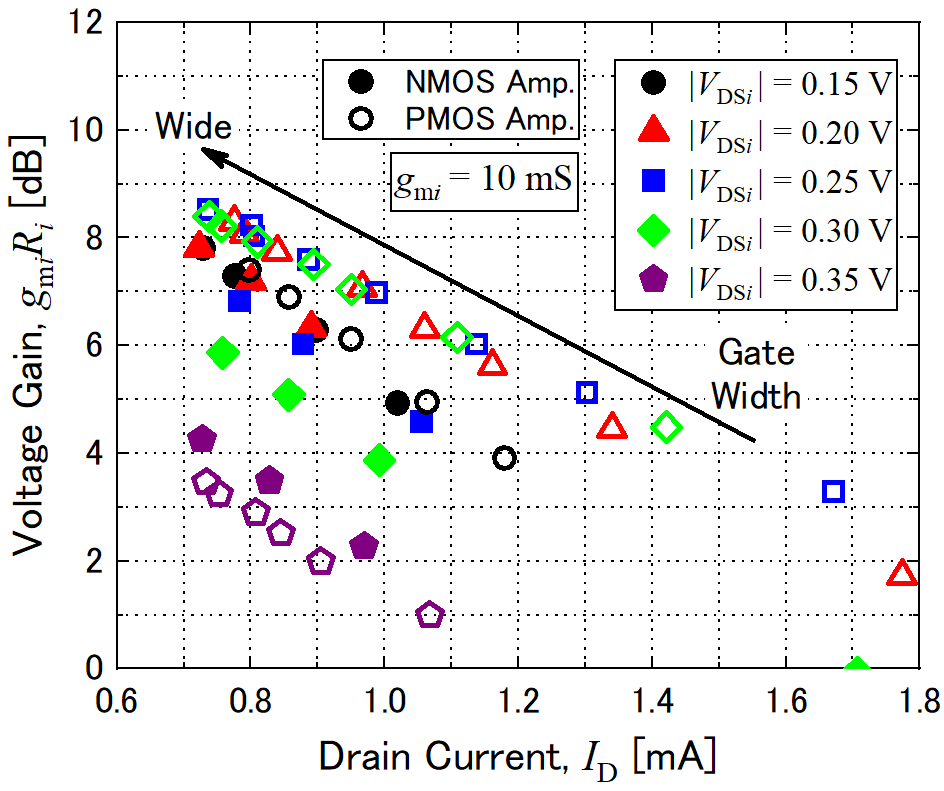Fig. 4. Dependence of voltage gain, $\textit{g}$$_{\mathrm{m}}$$_{i}$$\textit{R}$$_{i}$, upon drain current for case (ii): $\textit{V}$$_{\mathrm{DD1}} = 0.6 V, \textit{V}$$_{\mathrm{DD2}}$ = 0.6 V.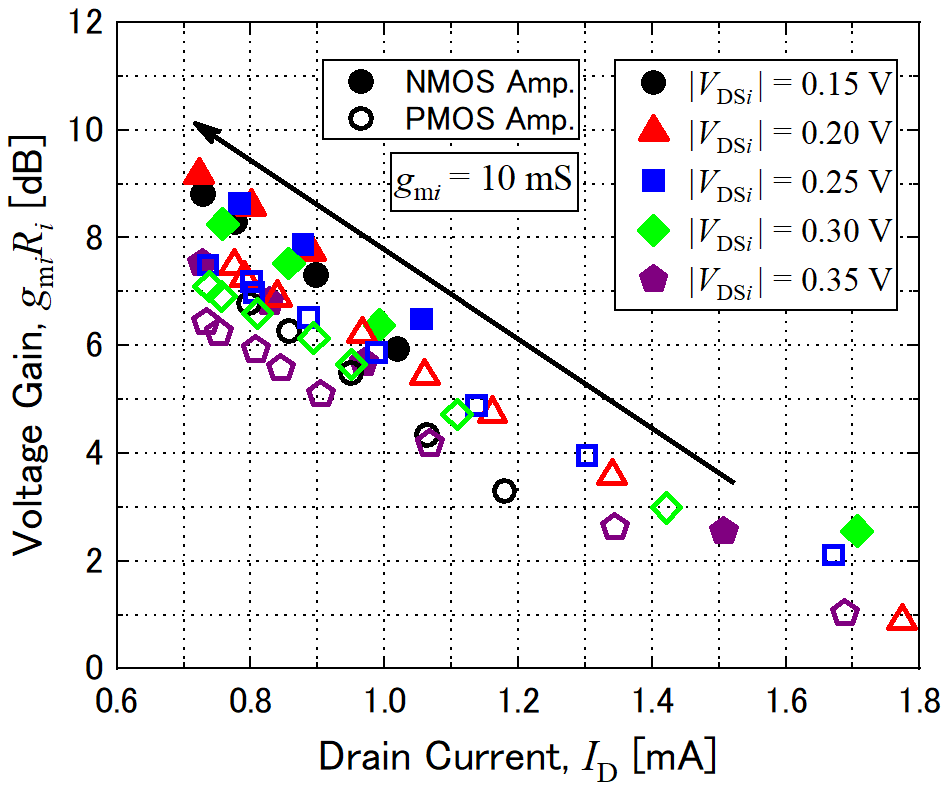We examined the characteristics of the stacked structure shown in Fig. 2(a). Based on the analyses shown in Figs. 3-5, the parameters of $\textit{V}$$_{\mathrm{GS}}$$_{i}$, $\textit{R}$$_{i}, and the gate width of M_{i} were chosen so that the NMOS and PMOS amplifiers could draw almost the same current. Then, the NMOS and PMOS amplifiers using the obtained parameters were stacked. Here, the intermediate voltage, \textit{V}$$_{\mathrm{MID}}$, is almost as same as $\textit{V}$$_{\mathrm{DD2}}. The drain current was set to 0.8 mA to obtain higher gain and operation in 2.4-GHz band. The load capacitance of the amplifier was set to 100 fF. Fig. 6 shows the voltage gains, \textit{v}$$_{\mathrm{out}}$$_{i}$$_{\mathrm{/}}$$\textit{v}$$_{\mathrm{in}}$, against the frequency for each intermediate-node voltage, where the solid and dashed lines signify $\textit{v}$$_{\mathrm{out1/}}$$\textit{v}$$_{\mathrm{in}} (NMOS amplifier side) and \textit{v}$$_{\mathrm{out2/}}$$\textit{v}$$_{\mathrm{in}}$ (PMOS amplifier side), respectively; the blue, red, and green lines signify the results of $\textit{V}$$_{\mathrm{MID}} = 0.7, 0.6, 0.5 V, respectively. The intermediate-node voltages, \textit{V}$$_{\mathrm{MID}}$ = 0.7, 0.6, 0.5 V, correspond to the cases (i), (ii), and (iii) in the previous subsection, respectively. As shown in Fig. 6, the magnitude relation between the gains of the NMOS and PMOS amplifiers has good agreement with that of the results shown in Figs. 3-5. The obtained bandwidth is around 3 GHz, which is enough for the 2.4-GHz-band applications.

Fig. 5. Dependence of voltage gain, $\textit{g}$$_{\mathrm{m}}$$_{i}$$\textit{R}$$_{i}$, upon drain current for case (iii): $\textit{V}$$_{\mathrm{DD1}} = 0.7 V, \textit{V}$$_{\mathrm{DD2}}$ = 0.5 V.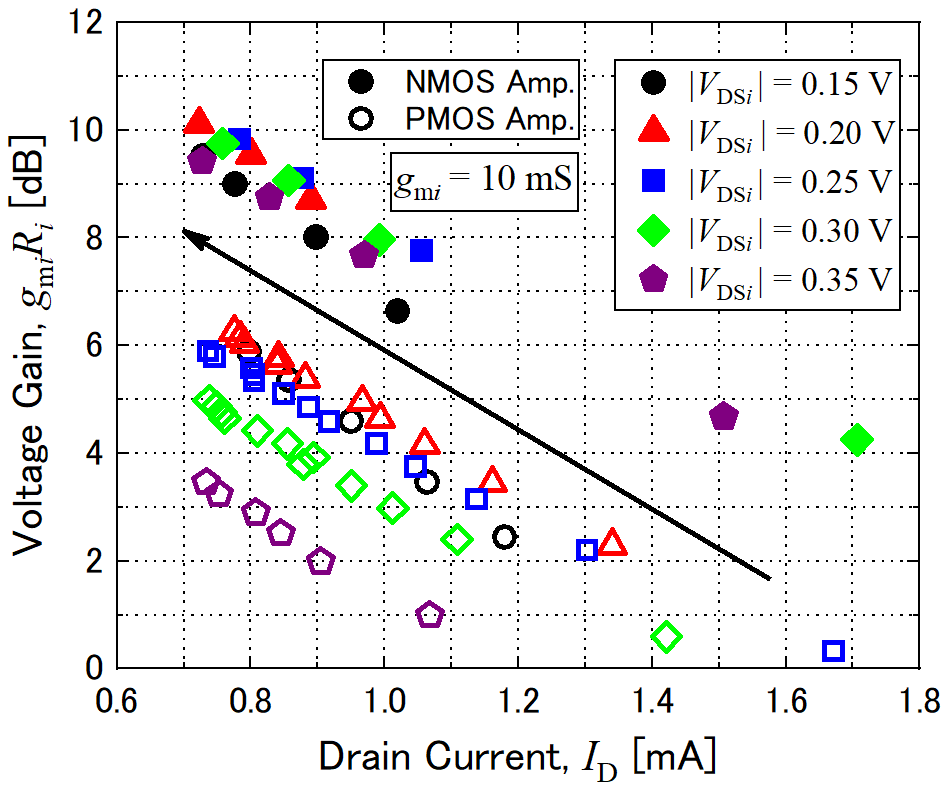Fig. 6. Voltage gain, $\textit{v}$$_{\mathrm{out}}$$_{i}$/$\textit{v}$$_{\mathrm{in}}, against frequency after stacking NMOS and PMOS amplifiers.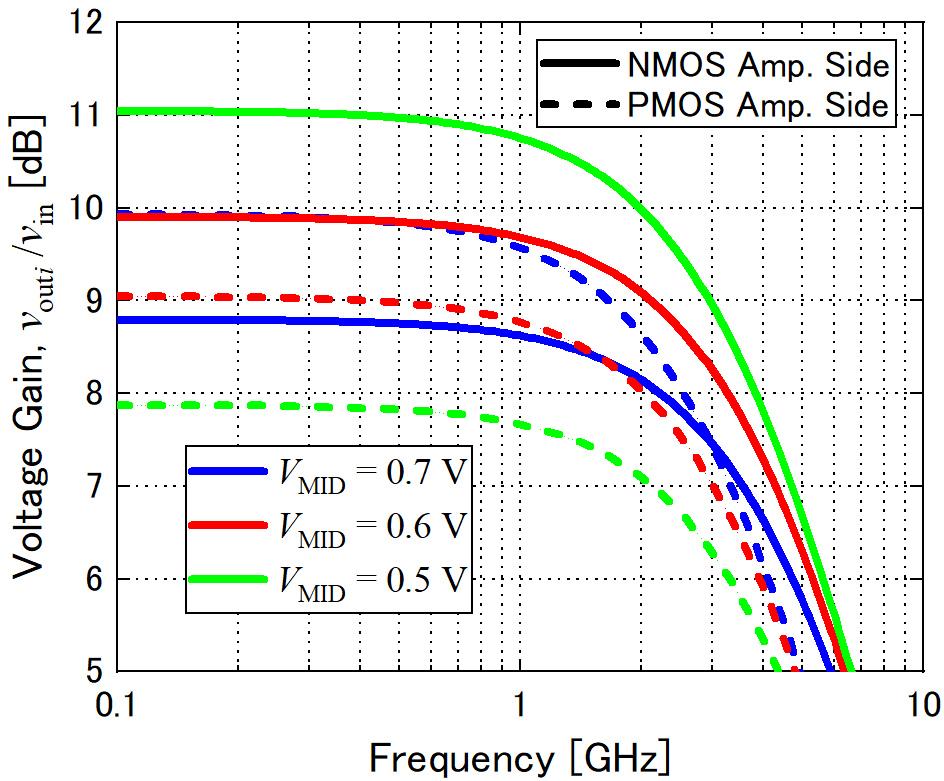As shown in Fig. 7(a), we add a feedback transistor (M_{3}) to the previous structure. The gate terminal of the feedback transistor is connected to the output terminal of the PMOS amplifier, and the transistor draws feedback current from the intermediate node. In this structure, the feedback current is reused by the NMOS amplifier, therefore it leads to reducing power consumption. The input impedance can be rewritten by ##### (5) Z_{\mathrm{in}}=\left\{\frac{g_{\mathrm{m}1}+g_{\mathrm{o}1}}{1+g_{\mathrm{o}1}R_{1}}+\frac{g_{\mathrm{m}2}+g_{\mathrm{o}2}}{1+g_{\mathrm{o}2}R_{2}}+\frac{g_{\mathrm{m}3}R_{2}\left(g_{\mathrm{m}2}+g_{\mathrm{o}2}\right)}{1+g_{\mathrm{o}2}R_{2}}+g_{\mathrm{o}3}\right\}^{- 1}, where \textit{g}$$_{\mathrm{m3}}$ and $\textit{g}$$_{\mathrm{o3}} are the transconductance and output conductance of the feedback transistor, respectively. In comparison with Eq. (2), the input impedance can be decreased by the effect of the feedback transistor as shown in the third and fourth terms of Eq. (5). Fig. 7. Schematic diagram after adding feedback transistor and coupling capacitor to basic structure.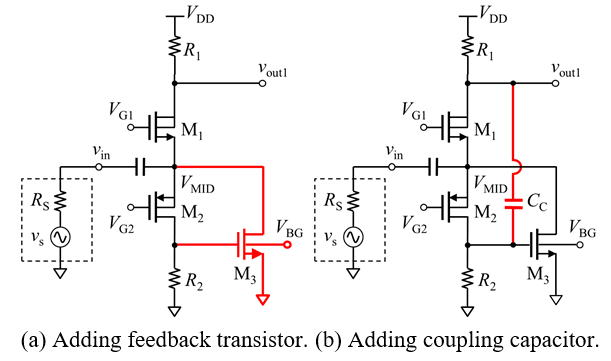Fig. 8. Frequency response of input impedance. Dashed and solid lines signify results without and with feedback, respectively.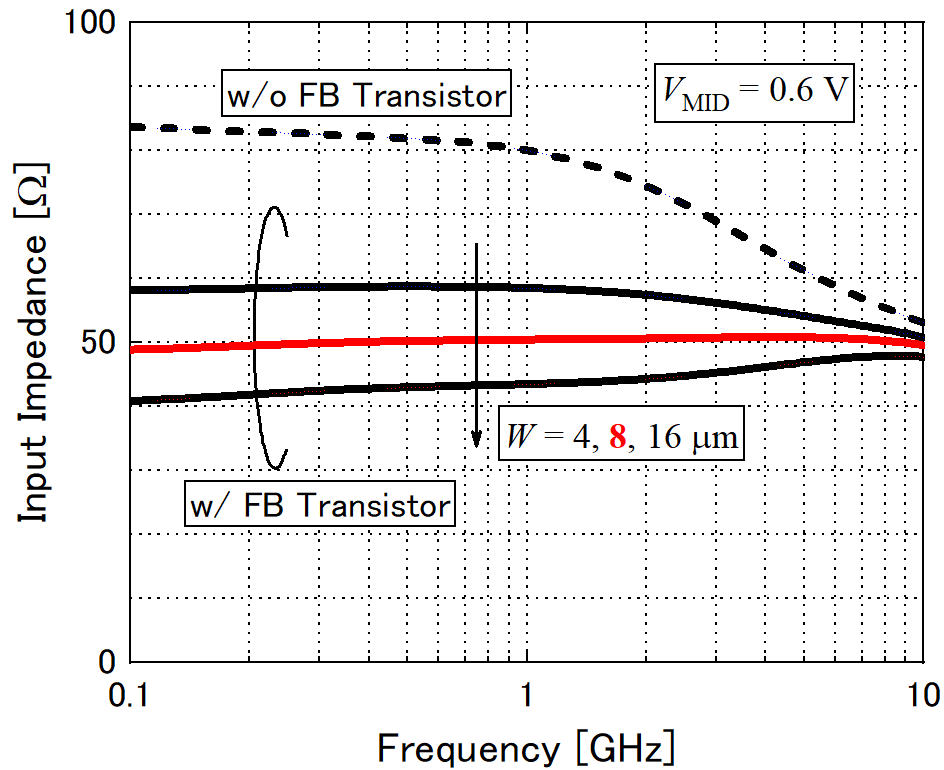Fig. 8 shows the frequency response of the input impedance in the case (ii) (\textit{V}$$_{\mathrm{MID}}$ = 0.6 V), where $\textit{W}$ is the gate width of the feedback transistor, dashed line and solid lines signify the results without and with the feedback, respectively. Although the impedance without the feedback is slightly higher than the matching condition, the matching condition can be satisfied by adding the transistor with appropriate gate width ($\textit{W}$ = 8~μm). The intermediate voltage corresponds to the gate voltage of M$_{3}$, the gate width of the case (i) or (iii) should be smaller or larger than that of the case (ii) to satisfy the matching condition, respectively.

Fig. 9. NF and $\textit{S}$$_{21} against coupling capacitance. Solid and dashed lines signify results of NF and \textit{S}$$_{21}$, respectively.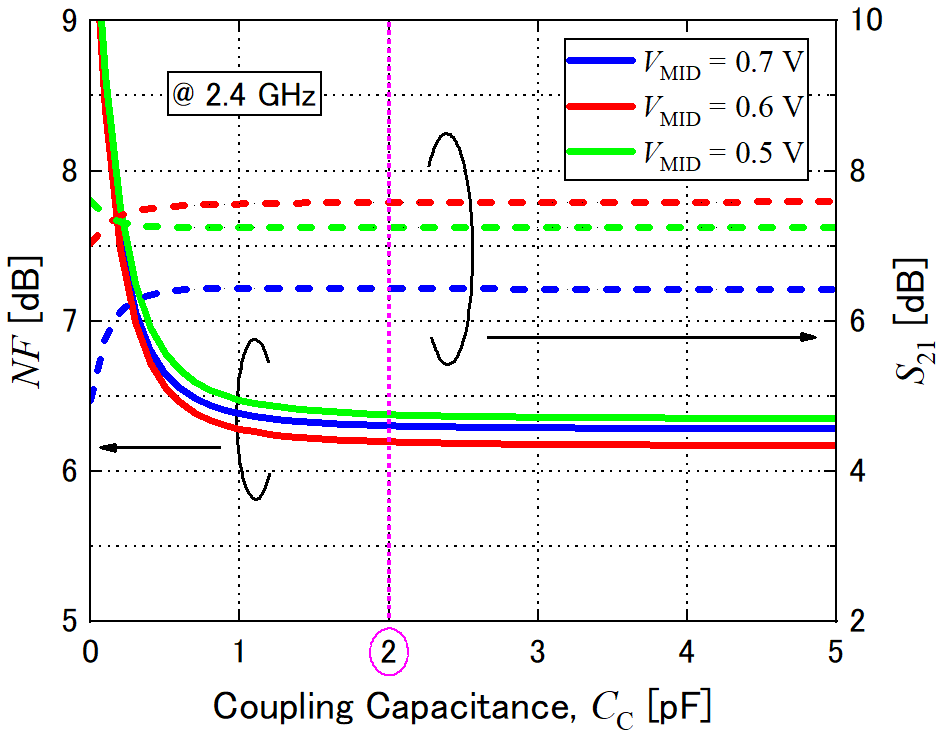Fig. 10. Frequency response of NF. Dashed and solid lines signify results without and with capacitor of 2 pF, respectively.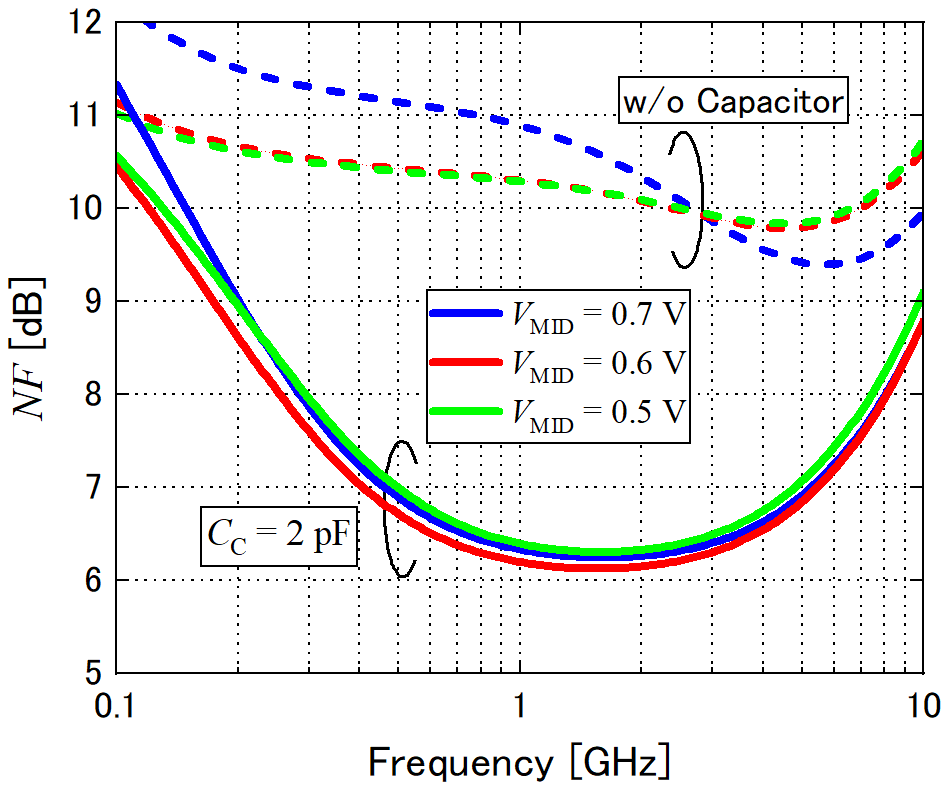### 4. Adding Coupling Capacitor and Examining Noise-Cancelling Effect

As shown in Fig. 7(b), we add a coupling capacitor ($\textit{C}$$_{\mathrm{C}}) to the basic structure. To evaluate \textit{S}-parameters and noise figure (NF), a buffer circuit (CS amplifier) with a 0-dB gain for the output matching is connected in this simulation. Fig. 9 shows the simulation results of NF (solid lines) and \textit{S}$$_{21}$ (dashed lines) against the coupling capacitance for each $\textit{V}$$_{\mathrm{MID}} at 2.4 GHz, where the blue, red, and green lines signify the results of \textit{V}$$_{\mathrm{MID}}$ = 0.7, 0.6, 0.5 V, respectively. The NF is reduced as the capacitance is increased. Although larger capacitance is required to obtain lower NF, increasing the capacitance results in occupying large chip area. Then, we chose 2 pF from the viewpoint of both reducing NF and saving chip area.

Fig. 10 shows the frequency response of NF without the capacitor (dashed lines) and with the capacitor (solid lines). Although the NF without the coupling capacitor was around 10 dB, the obtained NF was reduced to around 6 dB by adding the capacitor of 2 pF. As shown in Fig. 10, lower NF can be achieved by setting $\textit{V}$$_{\mathrm{MID}} = 0.6 V over the entire frequency band. ### 5. Effect of Back-gate Voltage Fig. 11. Dependences of \textit{S}-parameters upon back-gate voltage, \textit{V}$$_{\mathrm{BG}}$. Solid and dashed lines signify results of $\textit{S}$$_{11} and \textit{S}$$_{21}$, respectively.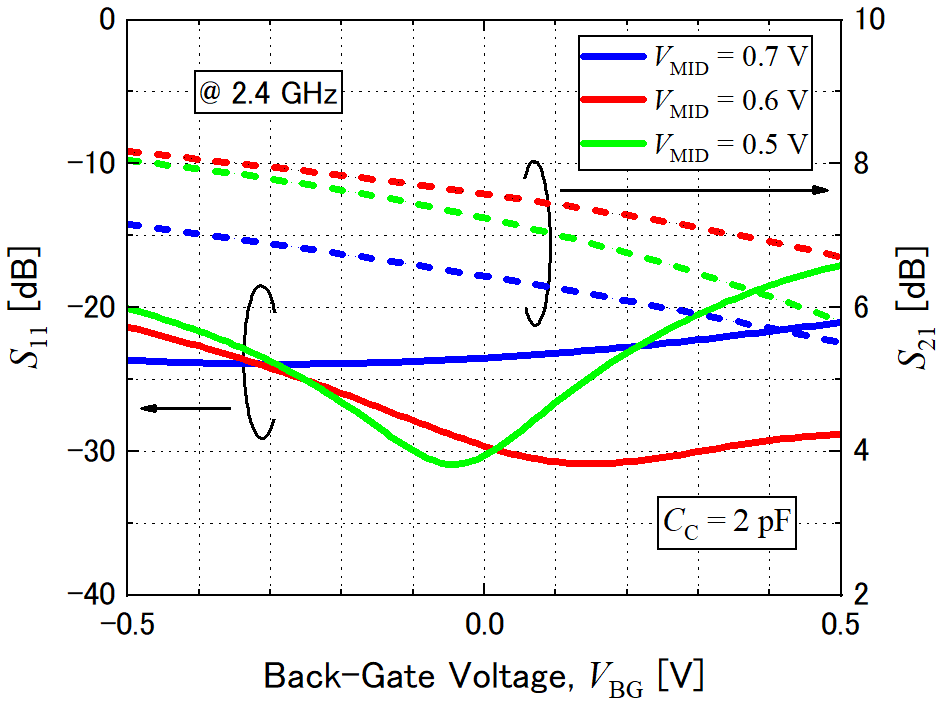Fig. 12. Dependences of NF upon back-gate voltage, $\textit{V}$$_{\mathrm{BG}}.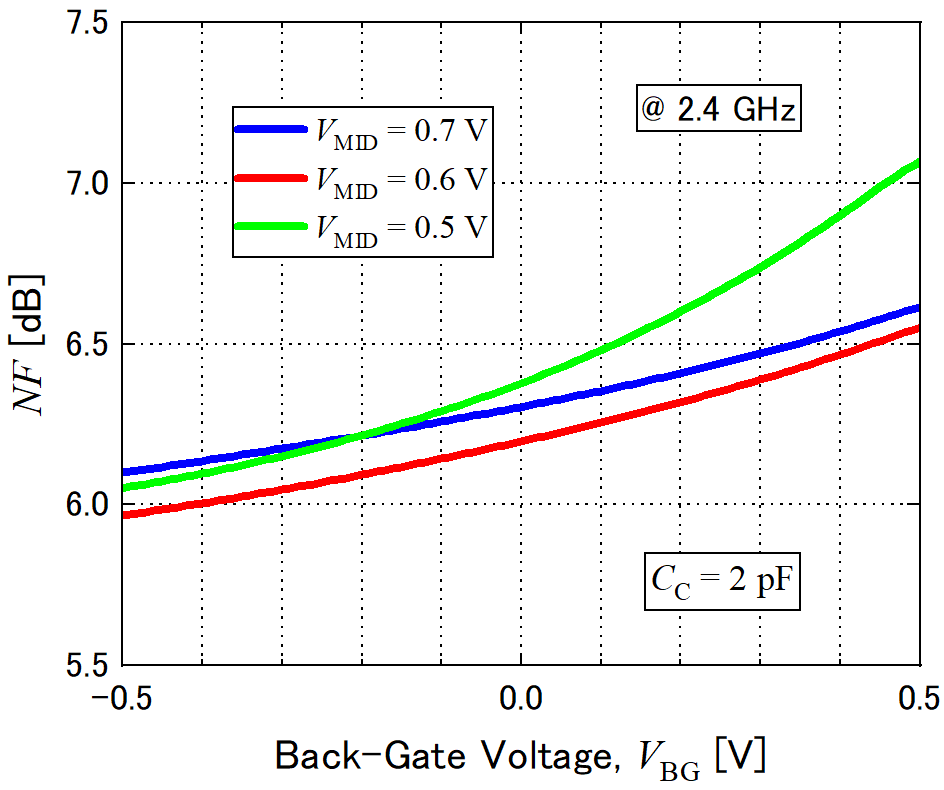Fig. 13. Linearity of proposed LNA. Solid and dashed lines signify fundamental and IM3 powers, respectively.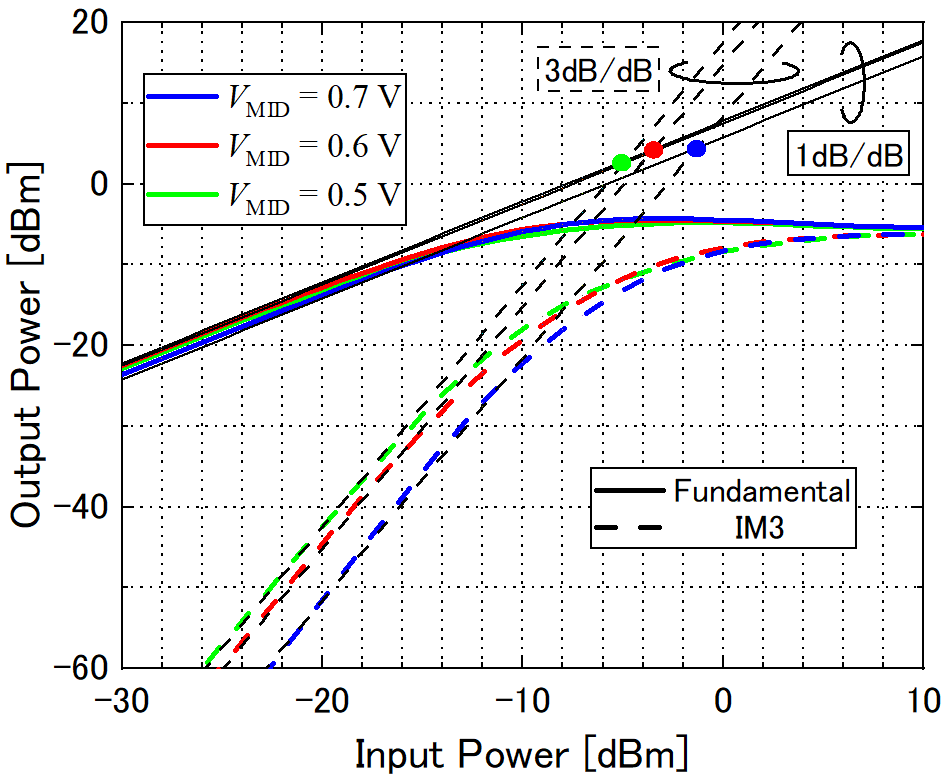We examined the effect of the back-gate voltage of the feedback transistor (M_{3}). The simulation results of \textit{S}-parameters against the back-gate voltage are shown in Fig. 11, where solid and dashed lines signify the results of \textit{S}$$_{11}$ and $\textit{S}$$_{21}, respectively. As shown in Fig. 11, the value of \textit{S}$$_{11}$ can be minimized by adjusting the back-gate voltage. The value of $\textit{S}$$_{21} is increased as the back-gate voltage is decreased, it reaches ~8 dB under \textit{S}$$_{11}$ < ${-}$20 dB at $\textit{V}$$_{\mathrm{BG}} = {-}0.5 V in the case (ii). Therefore, we can adjust the input impedance or the gain by changing the back-gate voltage. We also examined the effect of the back-gate voltage upon the noise characteristics. Fig. 12 shows the simulation results of NF against \textit{V}$$_{\mathrm{BG}}$ for $\textit{V}$$_{\mathrm{MID}} = 0.7, 0.6, 0.5 V. As shown in Fig. 11 and 12, lower NF is obtained by decreasing \textit{V}$$_{\mathrm{BG}}$ although $\textit{S}$$_{11} gets worse. This is because the noise matching condition is slightly different from the impedance matching condition. ### 6. Linearity Characteristics We examined the linearity of the proposed LNA by calculating the fundamental and third order intermodulated (IM3) component powers around 2.4 GHz. Fig. 13 shows the output fundamental and IM3 component powers against input power. This result shows that the highest input-referred third-order intercept point (IIP3) is obtained in the case of \textit{V}$$_{\mathrm{MID}}$ = 0.7 V. However, the gain ($\textit{S}$$_{21}) and NF for \textit{V}$$_{\mathrm{MID}}$ = 0.6 V are better than that for $\textit{V}$$_{\mathrm{MID}} = 0.7 V as shown in Fig. 9. Also, the output-referred IP3 (OIP3) of \textit{V}$$_{\mathrm{MID}}$ = 0.6 V is same as large as that of $\textit{V}$$_{\mathrm{MID}} = 0.7 V. ## IV. MEASUREMENT RESULTS We designed and fabricated the LNA using the TSMC 65-nm process on the basis of the proposed method. We chose the case (ii) for high gain and low NF, and readjusted the component values after post-layout simulation (PLS) as shown in Table 1. A die photograph of the fabricated LNA is shown in Fig. 14. The designed circuit consists of an LNA core circuit and a buffer circuit (0-dB gain) for output-impedance matching. The LNA occupies the area of 129 {\times} 92 μm^{2}. The \textit{S}-parameters of the LNA were measured by a vector network analyzer. Fig. 15 shows the PLS and measured results of \textit{S}$$_{11}$ and $\textit{S}$$_{21}. As shown in Fig. 15, the measured value of \textit{S}$$_{21}$ had good agreement with the PLS value. The frequency bandwidth (BW) was around 3 GHz, which is sufficient for 2.4-GHz-band applications. The measured $\textit{S}$$_{11} shows good reflection characteristics (< {-}10 dB) over the entire measurement range. The effect of applying the back-gate voltage to the feedback transistor (M_{3}) was examined. Fig. 14 shows the effect of the applied voltage \textit{V}$$_{\mathrm{BG}}$ on $\textit{S}$$_{11} and \textit{S}$$_{21}$. As shown in Fig. 16, the $\textit{S}$$_{11} and \textit{S}$$_{21 }$can be changed by applying externally controlled voltage. Thus, the feedback transistor can work to compensate for the divergence of the matching condition derived from the process variation.

Table 1. Device dimension and component values

 Gate Width / Length Resistance, Capacitance M1 32 μm / 60 nm R1 400$\Omega$ M2 64 μm / 60 nm R2 400$\Omega$ M3 8 μm / 60 nm Cc 2 pF

Fig. 15. Dependences of $\textit{S}$-parameters of fabricated LNA upon frequency. Solid and chain lines signify measured and PLS results, respectively.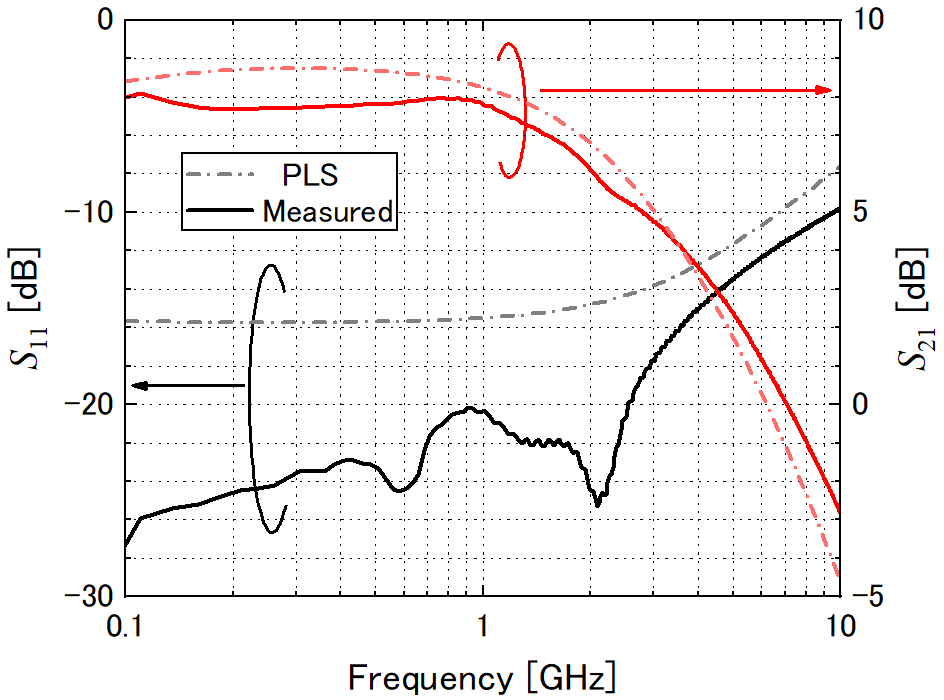### ACKNOWLEDGMENTS

This work is supported by Grants-in-Aid for Scientific Research, Japan Society for the Promotion of Science (JSPS). This work is also supported by VLSI Design and Education Center (VDEC), the University of Tokyo in collaboration with Cadence Design Systems, Inc. and Synopsys, Inc.

### REFERENCES

1
Wu T., Wu F., Redouté J.-M., Yuce M.R., 2017, An autonomous wireless body area network implementation towards IoT connected healthcare applications, IEEE Access, Vol. 5, No. , pp. 11413-114222
Oliveira L.M.L., Rodrigues J.J.P.C., 2011, Wireless sensor networks: a survey on environmental monitoring, J. Communications, Vol. 6, No. 2, pp. 143-1513
Gupta D., 2015, Wireless sensor networks ‘future trends and latest research challenges’, IOSR J. Electronics and Communication Engineering (IOSR-JECE), Vol. 10, No. 2, ver. 2, pp. 41-464
Lee T., 2004, The Design of CMOS Radio-Frequency Integrated Circuits, Cambridge University Press5
Razavi B., 2011, RF Microelectronics, 2nd ed. Prentice-Hall6
Bruccoleri F., Klumperink E. A. M., Nauta B., February 2004, Wide-band CMOS low-noise amplifier exploiting thermal noise canceling, IEEE J. SolidState Circuits, Vol. 39, No. 2, pp. 275-2827
Perumana B. G., Zhan J.-H. C., Taylor S. S., Carlton B. R., Laskar J., May 2008, Resistive-feedback CMOS low-noise amplifiers for multiband applications, IEEE Trans. Microw. Theory Techn., Vol. 56, No. 5, pp. 1218-12258
Dharmiza D. N. S., Oturu M., Tanoi S., Ito H., Ishihara N., Masu K., 2012, An inverter-based wideband low-noise amplifier in 40 nm complementary metal oxide semiconductor, Jpn. J. Appl. Phys., Vol. 51, No. 4s, pp. 04de079
Belmas F., Hameau F., Fournier J.-M., May 2012, A low power inductorless LNA with double Gm enhancement in 130 nm CMOS, IEEE J. Solid- State Circuits, Vol. 47, No. 5, pp. 1094-110310
Lee H., Chung T., Seo H., Choi I., Kim B., January 2015, A wideband differential low-noise-amplifier with IM3 harmonics and noise canceling, IEEE Microw. Wireless Compon. Lett., Vol. 25, No. 1, pp. 46-4811
Parvizi M., Allidina K., El-Gamal M. N., June 2016, An ultra-low-power wideband inductorless CMOS LNA with tunable active shunt-feedback, IEEE Trans. Microw. Theory Techn., Vol. 64, No. 6, pp. 1843-185312
Liu H.-J., Zhang Z.-F., February 2017, An ultra-low power CMOS LNA for WPAN applications, IEEE Microw. Wireless Compon. Lett., Vol. 27, No. 2, pp. 174-17613
Yu Y., Kang K., Fan Y., Zhao C., Liu H., Wu Y., Ban Y. L., Yin W. Y., 2017, Analysis and design of inductorless wideband low-noise amplifier with noise cancellation technique, IEEE Access, Vol. 5, pp. 9389-939714
Pan Z., Qin C., Ye Z., Wang Y., Yu Z., January 2018, Wideband Inductorless Low-Power LNAs with Gm Enhancement and Noise-Cancellation, IEEE Transactions on Circuits and Systems I: Regular Papers, Vol. 65, No. 1, pp. 26-3815
Inoue T., Noguchi R., Tsuchiya A., Kishine K., Onodera H., August 2018, Low-power and high-linearity inductorless low-noise amplifiers with active-shunt-feedback in 65-nm CMOS technology, 2018 IEEE 61st International Midwest Symposium on Circuits and Systems (MWSCAS), pp. 751-754## Author

Toshiyuki Inoue received the B.S., M.S. and Ph.D. degrees in Electrical Electronic and Information Engi-neering from Osaka University, Osaka, Japan in 2010, 2012 and 2015, respectively.

He joined the Depart-ment of Electronic Systems Engineering, The University of Shiga Prefecture, in 2017, and has been an Assistant Professor since 2017.

His research interests include RF circuits for wireless communication, wireless sensor networks, radio-over-fiber technique and optoelectronics.

Dr. Inoue is a member of the Institute of Electronics, Information and Communication Engineers (IEICE) of Japan and the Japan Society of Applied Physics (JSAP).

He received the Paper Award in 2013 from IEICE.

Akira Tsuchiya received the B.E., M.E. and Ph.D. degrees in Commu-nications and Computer Engineering from Kyoto University, Kyoto, Japan, in 2001, 2003, and 2005, respectively.

Since 2005, he has been an Assistant Professor in the Department of Communications and Computer Engineering, Graduate School of Informatics, Kyoto University.

Since 2017, he has been an Associate Professor in the Department of Electronic Systems Engineering, the University of Shiga Prefecture, Shiga, Japan.

His research interest includes modeling and design of on-chip passive components of high-frequency CMOS, and high-speed analog circuit design.

He is a member of the IEEE, IEICE and IPSJ.

Kishine Keiji received the B.S., and M.S. degrees in engineering science from Kyoto University, Kyoto, Japan, and Ph.D. degree in informatics from Kyoto University, Kyoto, Japan, in 1990, 1992, and 2006, respectively.

In 1992, he joined the Electrical Communication Laboratories, Nippon Telegraph and Telephone Corporation (NTT), Tokyo, Japan.

He has been engaged in research and design of high-speed, low-power circuits for Gb/s LSIs using Si-bipolar transistors, with application to optical communication systems in NTT System Electronics Laboratories, Kanagawa, Japan.

From 1997, he has been worked on research and development of over Gb/s Clock and Data Recovery IC at Network Service Innovation Laboratory in NTT Network Innovation Laboratories, Kanagawa, Japan.

He worked at Ubiquitous Interface Laboratory in NTT Microsystems Integration Laboratories, Kanagawa, Japan. Since 2008, he had been an Associate Professor, and now he is a Professor with the school of engineering, the University of Shiga prefecture, Shiga, Japan.

Dr. Kishine is a member of the IEEE Solid-State Circuits Society (SSCS) and Circuits and Systems (CAS), the Institute of Electronics, Information and Communication Engineers (IEICE) of Japan, the Institute of Electrical Engineers of Japan (IEEJ).